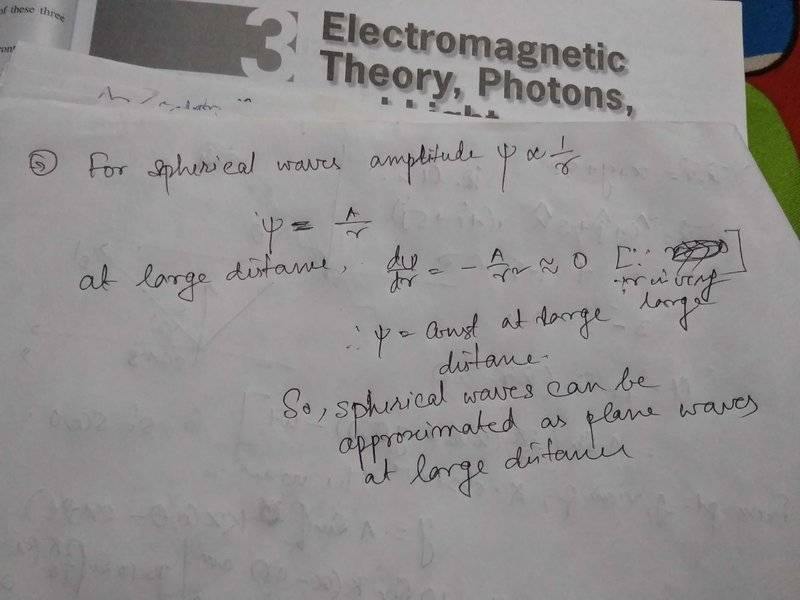# Are plane waves actually spherical waves at large distance?

• I
• Arup Biswas

#### Arup Biswas

I am doubting that any plane wave is generated from a spherical wave. At large distance the radii of curvature becomes so large that we can think it as plane. Like we se Earth surface as plane though it is spherical. Is it true? I have a mathematical proof for my argument!#### Attachments

At very large distances spherical waves are approximately plane waves (to first order)

At very large distances spherical waves are approximately plane waves (to first order)
Is the proof acceptable??

Is the proof acceptable??
I wouldn’t accept it as proof. It shows one characteristic, but doesn’t derive the equation for a plane wave.